# Matrices, NCERT Solutions, Class 12, Maths, Part -5 Notes - JEE

## JEE: Matrices, NCERT Solutions, Class 12, Maths, Part -5 Notes - JEE

The document Matrices, NCERT Solutions, Class 12, Maths, Part -5 Notes - JEE is a part of JEE category.
All you need of JEE at this link: JEE

Matrices

Miscellaneous Solutions

Question 1:
Let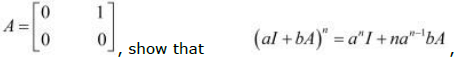where I is the identity
matrix of order 2 and n ε N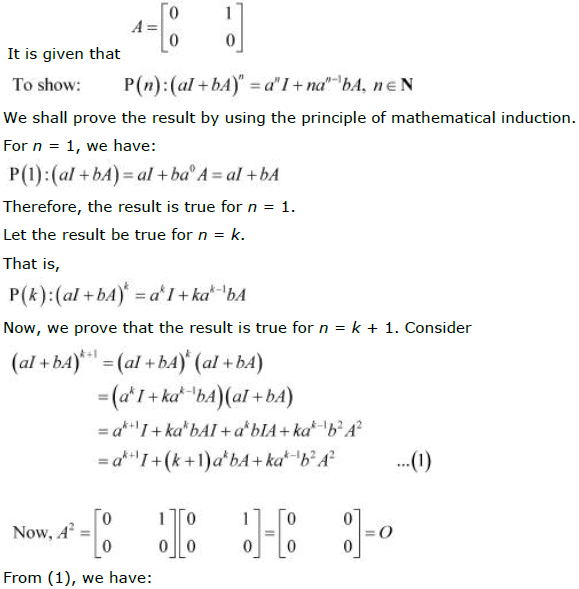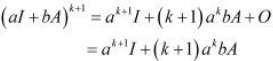Therefore, the result is true for n = k + 1.
Thus, by the principle of mathematical induction, we have: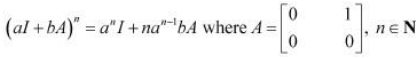Question 2: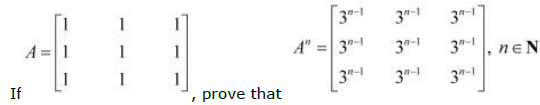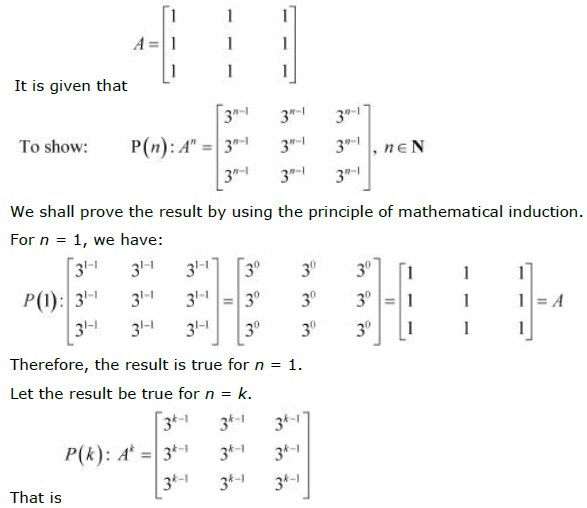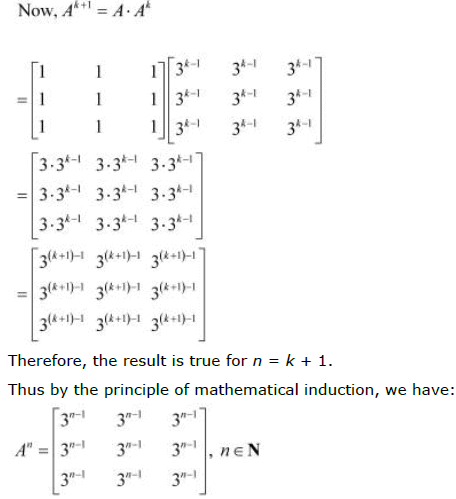Question 3: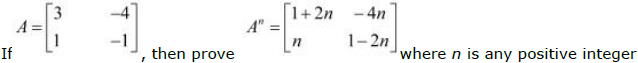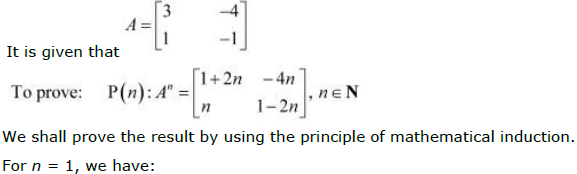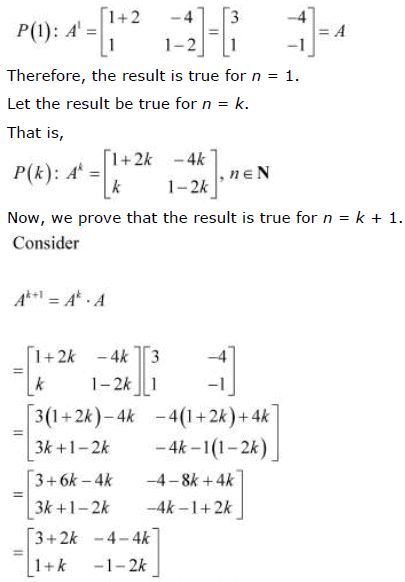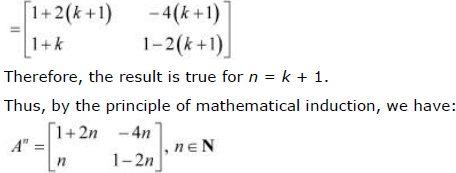Question 4:
If A and B are symmetric matrices, prove that AB − BA is a skew symmetric matrix.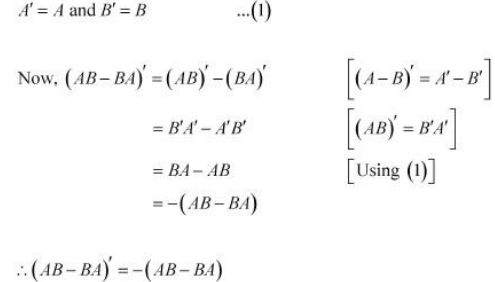Thus, (AB − BA) is a skew-symmetric matrix.

Question 5:
Show that the matrix B'AB is symmetric or skew symmetric according as A is symmetric
or skew symmetric.

We suppose that A is a symmetric matrix, then A' = A… (1)
Consider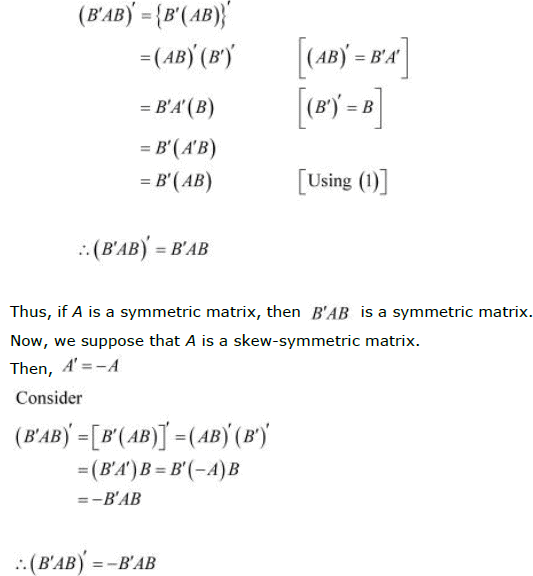Thus, if A is a skew-symmetric matrix, then B'AB is a skew-symmetric matrix.
Hence, if A is a symmetric or skew-symmetric matrix, then B'AB is a symmetric or
skewsymmetric matrix accordingly.

Question 6:
Solve system of linear equations, using matrix method.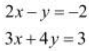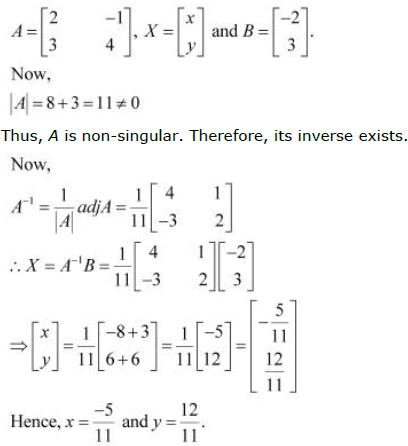Question 7:
For what values of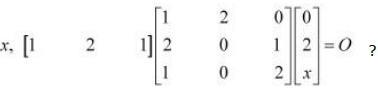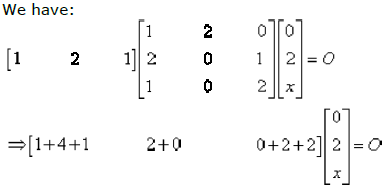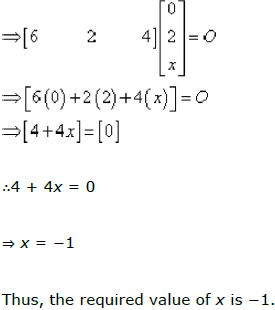Question 8:
If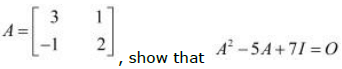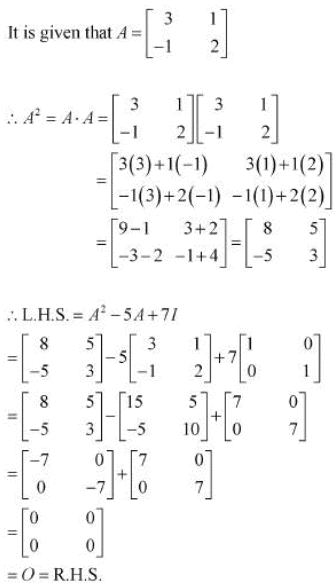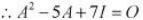Question 9:
Find x, if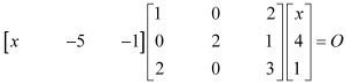We have: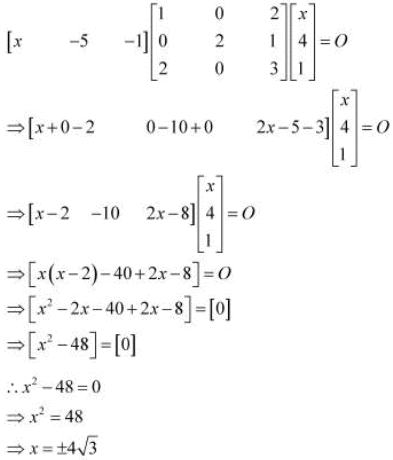Question 10:
A manufacturer produces three products x, y, z which he sells in two markets.
Annual sales are indicated below: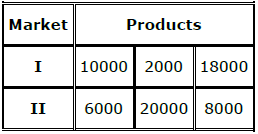(a) If unit sale prices of x, y and z are Rs 2.50, Rs 1.50 and Rs 1.00, respectively,
find the total revenue in each market with the help of matrix algebra.
(b) If the unit costs of the above three commodities are Rs 2.00, Rs 1.00 and 50
paise respectively. Find the gross profit.

(a) The unit sale prices of x, y, and z are respectively given as Rs 2.50, Rs 1.50, and Rs 1.00.
Consequently, the total revenue in market I can be represented in the form of a matrix
as: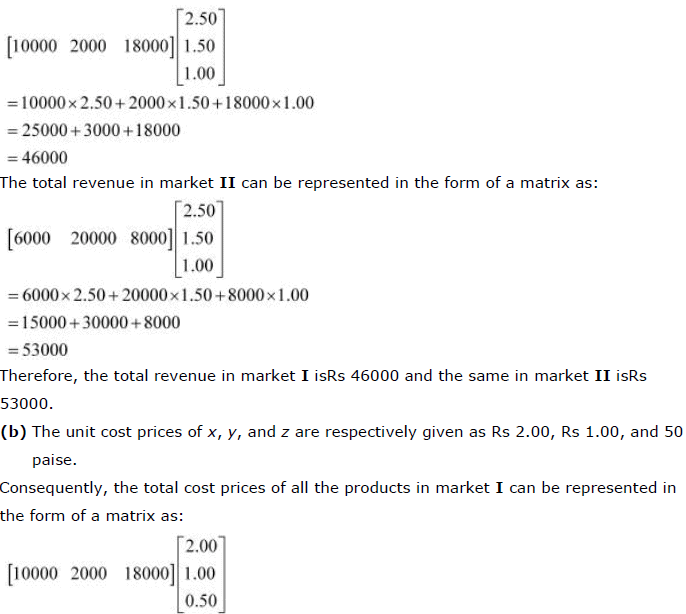The total revenue in market II can be represented in the form of a matrix as:
Therefore, the total revenue in market I isRs 46000 and the same in market II isRs
53000.
(b) The unit cost prices of x, y, and z are respectively given as Rs 2.00, Rs 1.00, and 50
paise.
Consequently, the total cost prices of all the products in market I can be represented in
the form of a matrix as: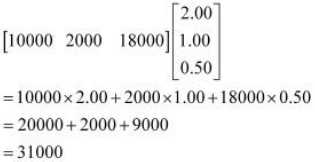Since the total revenue in market I isRs 46000, the gross profit in this marketis (Rs
46000 − Rs 31000) Rs 15000.
The total cost prices of all the products in market II can be represented in the form of a
matrix as: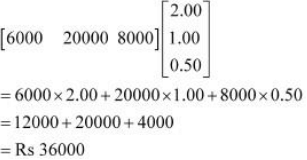Since the total revenue in market II isRs 53000, the gross profit in this market is (Rs
53000 − Rs 36000) Rs 17000.

Question 11:
Find the matrix X so that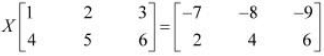It is given that:The matrix given on the R.H.S. of the equation is a 2 × 3 matrix and the one given on
the L.H.S. of the equation is a 2 × 3 matrix. Therefore, X has to be a 2 × 2 matrix.
Now, let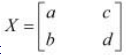Therefore, we have: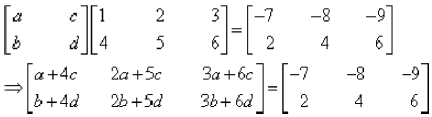Equating the corresponding elements of the two matrices, we have: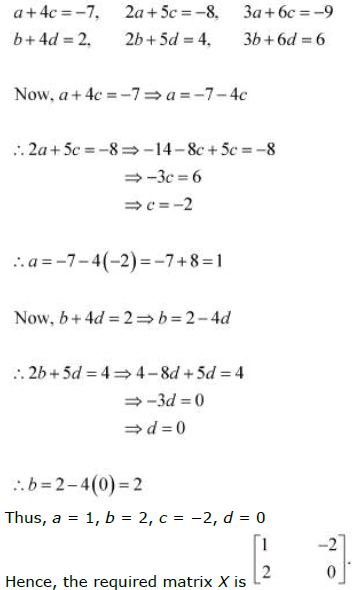Question 12:
If A and B are square matrices of the same order such that AB = BA, then prove by
induction that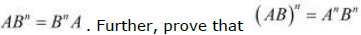for all n ε N

A and B are square matrices of the same order such that AB = BA.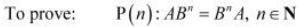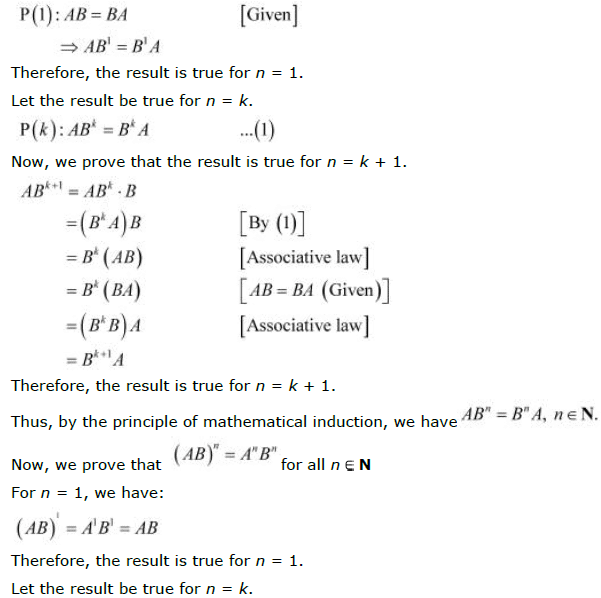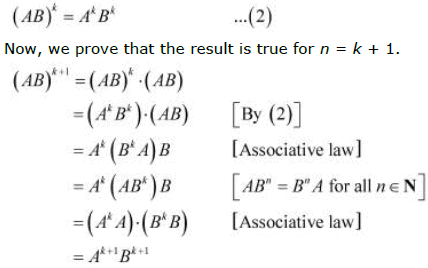Therefore, the result is true for n = k + 1.
Thus, by the principle of mathematical induction, we have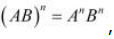, for all natural
numbers.

Question 13:
Choose the correct answer in the following questions: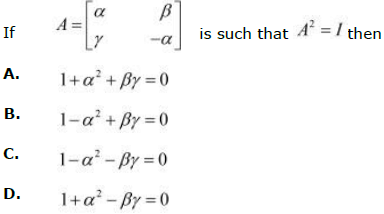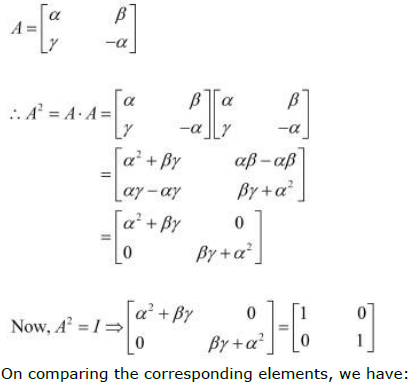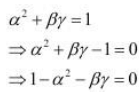Question 14:
If the matrix A is both symmetric and skew symmetric, then
A. A is a diagonal matrix
B. A is a zero matrix
C. A is a square matrix

If A is both symmetric and skew-symmetric matrix, then we should have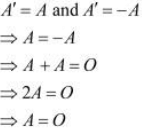Therefore, A is a zero matrix.

Question 15: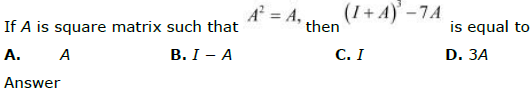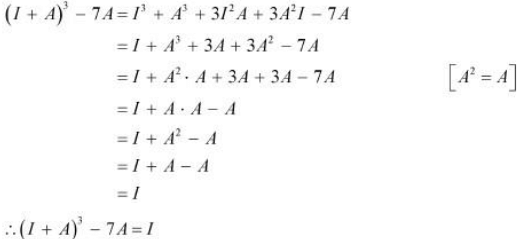The document Matrices, NCERT Solutions, Class 12, Maths, Part -5 Notes - JEE is a part of JEE category.
All you need of JEE at this link: JEEUse Code STAYHOME200 and get INR 200 additional OFF

Track your progress, build streaks, highlight & save important lessons and more!

,

,

,

,

,

,

,

,

,

,

,

,

,

,

,

,

,

,

,

,

,

,

,

,

,

,

,

,

,

,

,

,

,

;